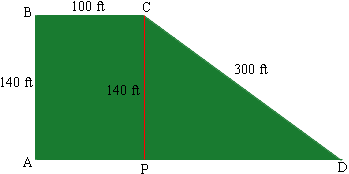Quandaries and Queries Question: I am trying to figure out how many square feet are in a piece of property. Start at Point A-then go 140 feet north-then 100 feet due east-then 300 feet at an angle southeast so that connecting to point A would be a straight line (right angle to first line north.) I sure hope you can understand this description. I'd really appreciate it if you could tell me HOW you figure something like this. Thanks. Deb Hi Deb, I drew a diagram of you lot and added a perpendicular line from C to DA meeting DA at P to see that the lot can be divided into a rectangle APCB and a right triangle DCP.The rectangular section has area 100140 = 14 000 square feet. The area of a triangle is half the base times the height so I can calculate the area of the triangle if I can find the length of the base PD. I can find this using Pythagoras' Theorem. (It is really amazing how useful this theorem is.) Pythagoras' Theorem for triangle DCP says |CP|2 + |PD|2 = |DC|2 That is 1402 + |PD|2 = 3002 so |PD| = Sqrt[3002 - 1402] = Sqrt = 265.33 ft Hence the area of the triangle is  1/2|PD||CP| = 1/2265.33140 = 18 573 square feet. Thus the area of your property is 14 000 + 18 573 = 32 573 square feet. Penny Go to Math Central